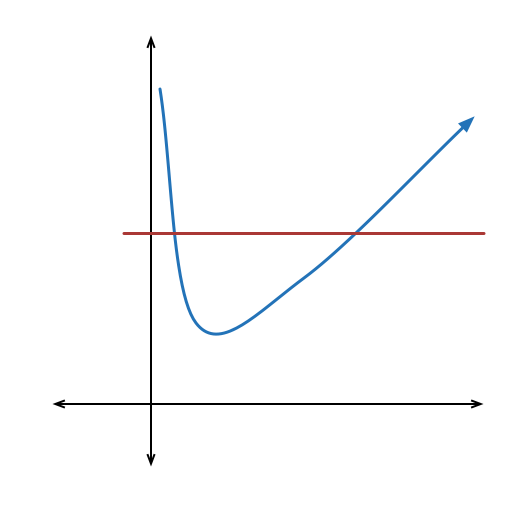# Natural selection!

Probability Level 3A natural number $x$ is selected from the set of the first $100$ positive integers. Find the probability that it satisfies the inequality: $x + \dfrac{100}{x} > 50$ If the probability is of the form $\dfrac{a}{b}$ , where a and b are coprime integers, find the value of $a + b$.

×